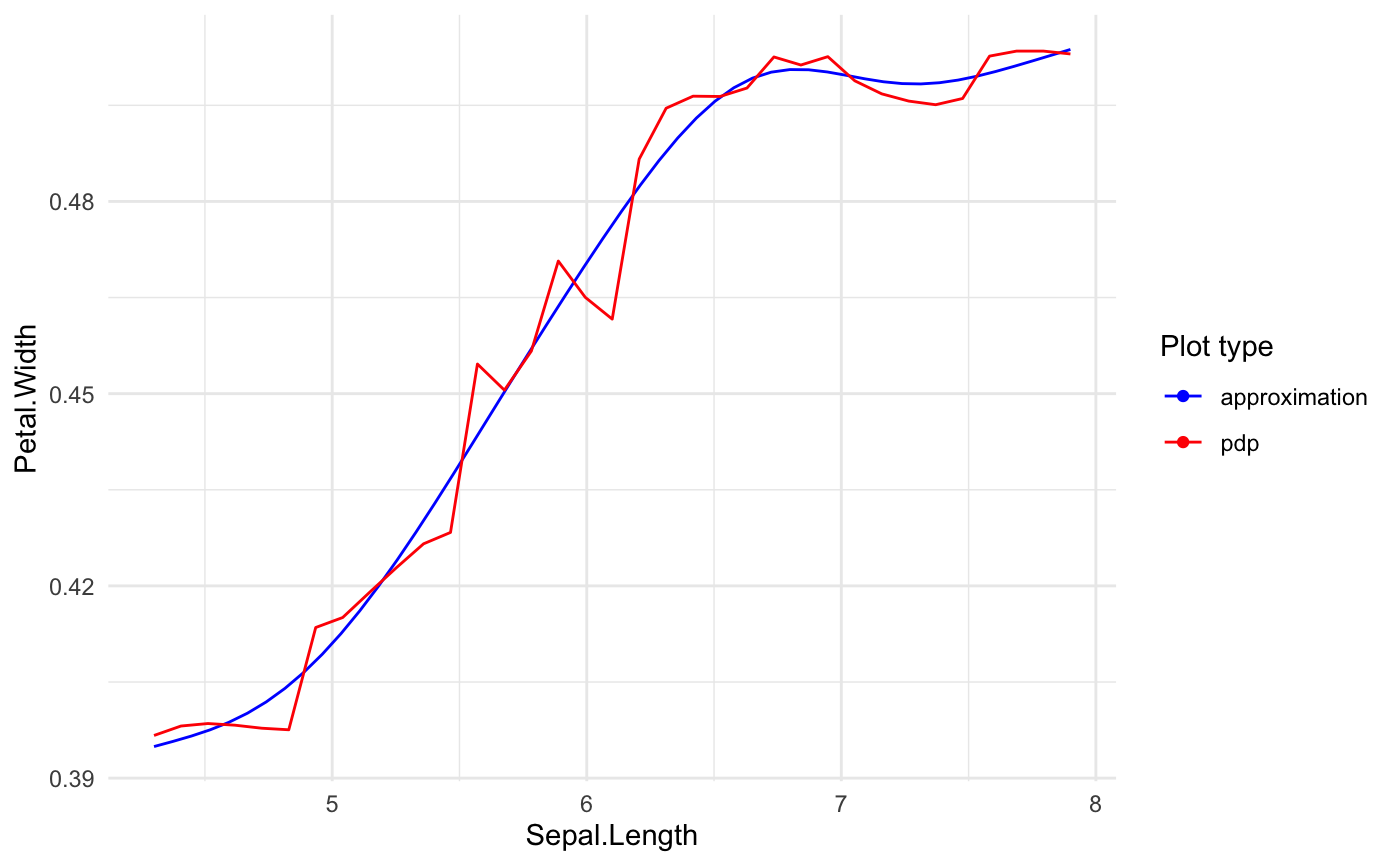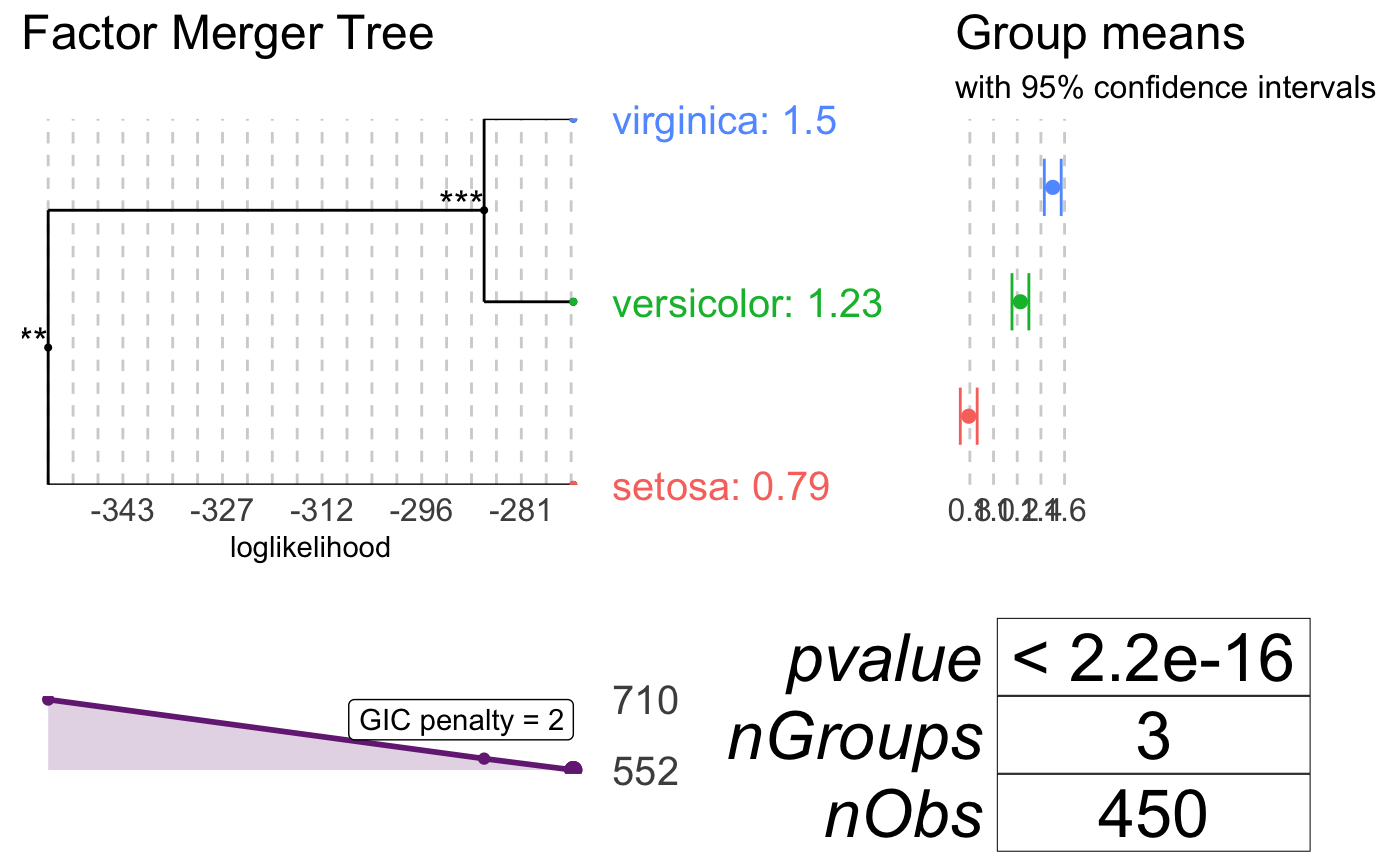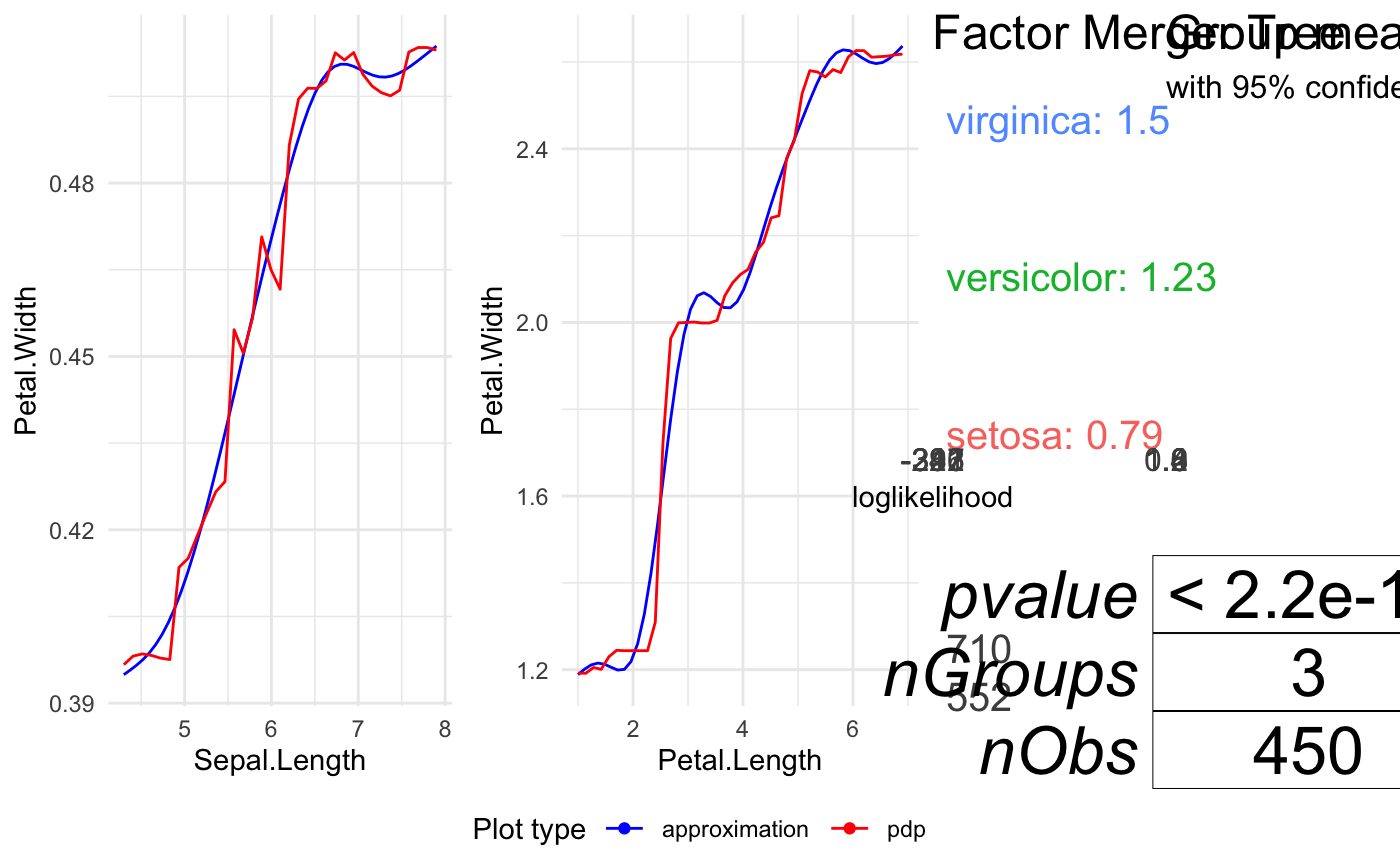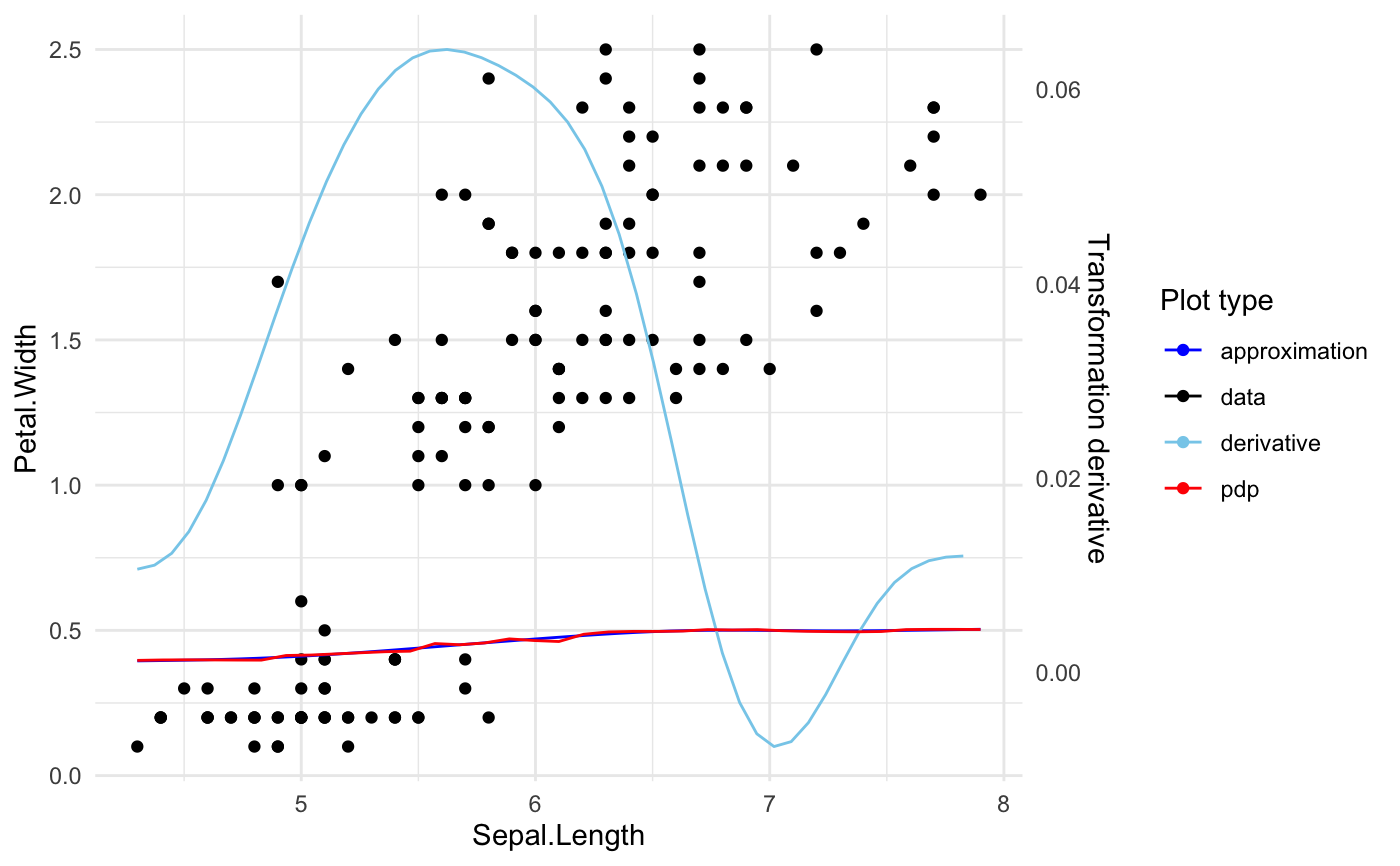The function plots variable profile. In case of quantiitative variable it plots original transition function and its spline approximation. The function provides possibility to plot data points and transition derivative as well. In case of qualitative variable it plots merging path for variable levels. When no variable is specified it plots transitions for first n_plots variables.

plot_variable_transition(x, variable_names = NULL,
plot_response = TRUE, plot_approx = TRUE, data = NULL,
plot_data = FALSE, plot_deriv = FALSE, n_plots = 6,
use_coeff = TRUE)

## Arguments

x Object of class 'xspliner'. Names of predictors which transitions should be plotted. If TRUE black box model response is drawn. If TRUE black box model response approximation is drawn. Training data used for building x model. Required for plot_data option. If TRUE raw data is drawn. If TRUE derivative of approximation is showed on plot. Threshold for number of plots when plotting all variables. If TRUE both PDP function and its approximation is scaled with corresponding surrogate model coefficient.

## Examples

library(randomForest)
set.seed(1)
data <- iris
# regression model
iris.rf <- randomForest(Petal.Width ~  Sepal.Length + Petal.Length + Species, data = data)
iris.xs <- xspline(iris.rf)
# plot Sepal.Length transition
plot_variable_transition(iris.xs, "Sepal.Length")# plot Species transition
plot_variable_transition(iris.xs, "Species")# plot all transitions
plot_variable_transition(iris.xs)# plot Sepal.Length transition, its derivative and data points
plot_variable_transition(iris.xs, "Sepal.Length", data = data, plot_data = TRUE, plot_deriv = TRUE)